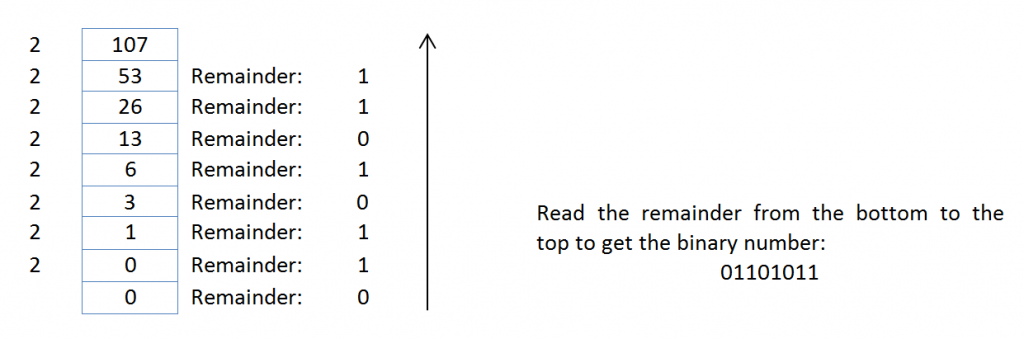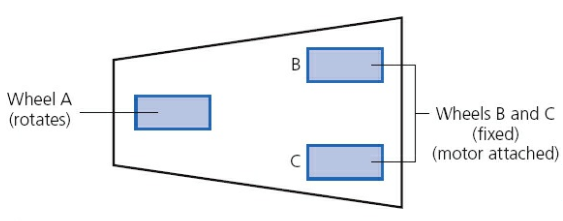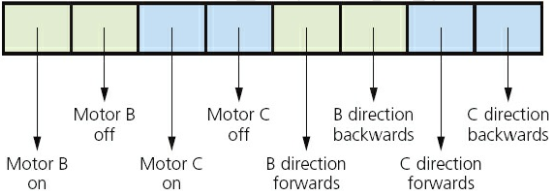# Understanding Binary

Watch out! This tutorial is over 5 years old. Please keep this in mind as some code snippets provided may no longer work or need modification to work on current systems.
Tutorial Difficulty Level

The basic building block in all computers is the binary number system.

This system is chosen since it consists of 1s and 0s only. Since computers contain millions and millions of tiny ‘switches’, which must be in the ON or OFF position, this lends itself logically to the binary system. A switch in the ON position can be represented by 1; a switch in the OFF position can be represented by 0.

We are all familiar with the denary (base 10) number system which counts in multiples of 10. This gives us the well-known headings of units, 10s, 100s, 1000s and so on:

 10000 1000 100 10 1 104 103 102 101 100

The binary system is based on the number 2. Thus, only the two ‘values’ 0 and 1 can be used in this system to represent each digit. Using the same method as denary, this gives the headings of  20,21,22,23 and so on. The typical headings for a binary number with eight digits would be:

 128 64 32 16 8 4 2 1 27 26 25 24 23 22 21 20

A typical binary number would be:

11101110

### Converting from binary to denary

It is fairly straightforward to change a binary number into a denary number. Each time a 1 appears in a column, the column value is added to the total. For example, the binary number above is:

128 + 64 + 32 + 8 + 4 + 2 = 238 (denary)

The 0 values are simply ignored.

### Converting from denary to binary

The reverse operation, converting from denary to binary, is slightly more complex. There are two basic ways of doing this. The first method is ‘trial and error’ and the second method is more methodical and involves repetitive division.

Method 1

Consider the conversion of the denary number, 107, into binary. This method involves placing 1s in the appropriate position so that the total equates to 107:

 128 64 32 16 8 4 2 1 0 1 1 0 1 0 1 1

Method 2

This method involves successive division by 2. The remainders are then read from BOTTOM to TOP to give the binary value. Again using 107, we get:### Example use of binary

Here is an example of using the binary system in a computer REGISTER. A register is a group of bits; it is often depicted as follows:

 1 0 0 1 0 1 1 1

When computers (or microprocessors) are used to control devices (such as robots), registers are used as part of the control system. The following example describes how registers can be used in controlling a simple device.

A robot vacuum cleaner has three wheels, A, B and C. A rotates on a spindle to allow for direction changes (as well as forward and backward movement); B and C are fixed to revolve around their axles to provide only forward and backward movement, and have an electric motor attached:An 8-bit register is used to control the movement of the robot vacuum cleaner:If the register contains 1 0 1 0 1 0 1 0 this means ‘motor B is ON and motor C is ON and both motors are turning to produce FORWARDS motion’. Effectively, the vacuum cleaner is moving forwards.

### Measurement of the size of computer memory

A binary digit is commonly referred to as a BIT; 8 bits are usually referred to as a BYTE.

The byte is the smallest unit of memory in a computer. Some computers use larger bytes but they are always multiples of 8 (e.g. 32-bit systems and 64-bit systems). One byte of memory wouldn’t allow you to store very much information; therefore memory size is measured in the following multiples:

 Name Number of bits Denary value 1 kilobyte (1KB) 210 1024 bytes 1 megabyte (1MB) 220 1048576 bytes 1 gigabyte (1GB) 230 1073741824 bytes 1 terabyte (1TB) 240 1099511627776 bytes 1 petabyte (1PB) 250 1125899906842624 bytes

To give some idea of the scale of these numbers, a typical data transfer rate using the internet is 32 megabits (i.e. 4 MB) per second (so a 40 MB file would take 10 seconds to transfer). Most hard disk systems in computers are 1 or 2 TB in size (so a 2 TB memory could store over half a million 4 MB photos, for example).

It should be pointed out here that there is some confusion in the naming of memory sizes. The IEC convention is now adopted by some organisations; however manufacturers of storage devices often use the denary system to measure storage size. For example,

1 kilobyte = 1000 byte

1 megabyte = 1000000 bytes

1 gigabyte = 1000000000 bytes

1 terabyte = 1000000000000 bytes and so on.

The IEC convention for computer internal memories (including RAM) becomes:

1 kibibyte (1 KiB) = 1024 bytes

1 mebibyte (1 MiB) = 1048576 bytes

1 gibibyte (1 GiB) = 1073741824 bytes

1 tebibyte (1 TiB) = 1099511627776 bytes and so on.